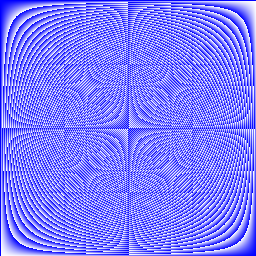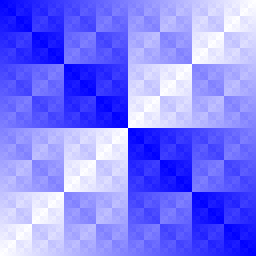# Go语言切片入门

 1  a[low : high] 

 1  a[1:4] 

  1 2 3 4 5 6 7 8 9 10  package main import "fmt" func main() { primes := int{2, 3, 5, 7, 11, 13} var s []int = primes[1:4] fmt.Println(s) } 

## 数组引用

  1 2 3 4 5 6 7 8 9 10 11 12 13 14 15 16 17 18 19 20 21  package main import "fmt" func main() { names := string{ "John", "Paul", "George", "Ringo", } fmt.Println(names) a := names[0:2] b := names[1:3] fmt.Println(a, b) b = "XXX" fmt.Println(a, b) fmt.Println(names) } 

## 下标默认值

 1  var a int 

 1 2 3 4  a[0:10] a[:10] a[0:] a[:] 

  1 2 3 4 5 6 7 8 9 10 11 12 13 14 15 16  package main import "fmt" func main() { s := []int{2, 3, 5, 7, 11, 13} s = s[1:4] fmt.Println(s) s = s[:2] fmt.Println(s) s = s[1:] fmt.Println(s) } 

## 长度和容量

  1 2 3 4 5 6 7 8 9 10 11 12 13 14 15 16 17 18 19 20 21 22 23 24 25  package main import "fmt" func main() { s := []int{2, 3, 5, 7, 11, 13} printSlice(s) // Slice the slice to give it zero length. s = s[:0] printSlice(s) // Extend its length. s = s[:4] printSlice(s) // Drop its first two values. s = s[2:] printSlice(s) } func printSlice(s []int) { fmt.Printf("len=%d cap=%d %v\n", len(s), cap(s), s) } 

## 空切片

  1 2 3 4 5 6 7 8 9 10 11  package main import "fmt" func main() { var s []int fmt.Println(s, len(s), cap(s)) if s == nil { fmt.Println("nil!") } } 

## make

make 函数分配一个由 零值 填充的数组，并返回一个引用该数组的切片：

 1  a := make([]int, 5) // len(a)=5 

 1 2 3 4  b := make([]int, 0, 5) // len(b)=0, cap(b)=5 b = b[:cap(b)] // len(b)=5, cap(b)=5 b = b[1:] // len(b)=4, cap(b)=4 

  1 2 3 4 5 6 7 8 9 10 11 12 13 14 15 16 17 18 19 20 21 22  package main import "fmt" func main() { a := make([]int, 5) printSlice("a", a) b := make([]int, 0, 5) printSlice("b", b) c := b[:2] printSlice("c", c) d := c[2:5] printSlice("d", d) } func printSlice(s string, x []int) { fmt.Printf("%s len=%d cap=%d %v\n", s, len(x), cap(x), x) } 

## 切片的切片

  1 2 3 4 5 6 7 8 9 10 11 12 13 14 15 16 17 18 19 20 21 22 23 24 25 26  package main import ( "fmt" "strings" ) func main() { // Create a tic-tac-toe board. board := [][]string{ []string{"-", "_", "_"}, []string{"-", "_", "_"}, []string{"-", "_", "_"}, } // The players take turns. board = "X" board = "O" board = "X" board = "O" board = "X" for i := 0; i < len(board); i++ { fmt.Printf("%s\n", strings.Join(board[i], " ")) } } 

## 元素追加

 1  func append(s []T, vs ...T) []T 

append 函数第一个参数 s 是一个类型为 T 的切片，其余参数均为追加至 sT 元素。

append 函数返回一个新切片，包含原切片以及所有追加元素。

  1 2 3 4 5 6 7 8 9 10 11 12 13 14 15 16 17 18 19 20 21 22 23 24  package main import "fmt" func main() { var s []int printSlice(s) // append works on nil slices. s = append(s, 0) printSlice(s) // The slice grows as needed. s = append(s, 1) printSlice(s) // We can add more than one elements at a time. s = append(s, 2, 3, 4) printSlice(s) } func printSlice(s []int) { fmt.Printf("len=%d cap=%d %v\n", len(s), cap(s), s) } 

## 遍历

  1 2 3 4 5 6 7 8 9 10 11  package main import "fmt" var pow = []int{1, 2, 4, 8, 16, 32, 64, 128} func main() { for i, v := range pow { fmt.Printf("2**%d=%d\n", i, v) } } 

  1 2 3 4 5 6 7 8 9 10 11 12 13 14 15  package main import "fmt" func main() { pow := make([]int, 10) for i := range pow { pow[i] = 1 << uint(i) // == 2**i } for _, value := range pow { fmt.Printf("%d\n", value) } } 

## 练习

### 1. 图片像素矩阵

  1 2 3 4 5 6 7 8 9 10  package main import "golang.org/x/tour/pic" func Pic(dx, dy int) [][]uint8 { } func main() { pic.Show(Pic) } 

• $\frac{x+y}{2}$

• $x \times y$

• $x^y$#### 答案

  1 2 3 4 5 6 7 8 9 10 11 12 13 14 15 16 17 18 19 20 21  package main import "golang.org/x/tour/pic" func Pic(dx, dy int) [][]uint8 { s := make([][]uint8, dy) for y := 0; y < dy; y++ { sx := make([]uint8, dx) for x := 0; x < dx; x++ { sx[x] = uint8((x+y)/2) //sx[x] = uint8(x*y) //sx[x] = uint8(x^y) } s[y] = sx } return s } func main() { pic.Show(Pic) } 

【小菜学Go语言】系列文章首发于公众号【小菜学编程】，敬请关注：【小菜学Go语言】系列文章首发于公众号【小菜学编程】，敬请关注：